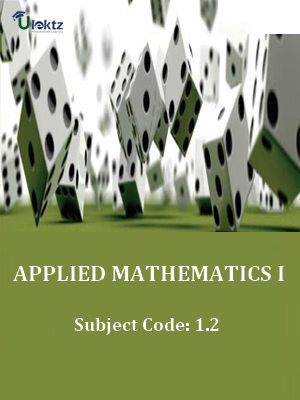•My WalletMy Order
•My Profile
•My Connections
•My Books
•My Videos
•My Tests
•My Calender
•My Messages
•My Shopping Cart
•My Orders
•Account Settings
•Help

# Book Details# Applied Mathematics-I

 Course Code : 1.2 Author : uLektz University : Haryana State Board of Technical Education Regulation : 2017 Categories : Electronics & Communication Format :ePUB3 (DRM Protected) Type : eBook

FREE

Description :Applied Mathematics-I of 1.2 covers the latest syllabus prescribed by Haryana State Board of Technical Education for regulation 2017. Author: uLektz, Published by uLektz Learning Solutions Private Limited.

Note : No printed book. Only ebook. Access eBook using uLektz apps for Android, iOS and Windows Desktop PC.

##### Topics
###### UNIT I ALGEBRA

1.1 Complex Numbers: definition of complex number, real and imaginary parts of a complex number. Polar and Cartesian Form and their inter conversion, Modulus and amplitude,Conjugate of a complex number. Addition subtraction, multiplication and division of complex number.

1.2 Logarithms and its basic properties.

1.3 Meaning of npr & ncr(mathematical expression). Binomial theorem (without proof) for positive integral index (expansion and general form). Binomial theorem for any index (expansion without proof), First binomial approximation with application to engineering problems.

1.4 Determinants and Matrices. Evaluation of determinants (up to 2ndorder), solution of equations (up to 2 unknowns) by Crammer’s rule. Definition of Matrices and its types, addition, subtraction and multiplication of matrices (up to 2ndorder)

###### UNIT II TRIGONOMETRY

2.1 Concept of angle, measurement of angle in degrees, grades, radians and their conversions.

2.2 T-Ratios of Allied angles (without proof), (Transformation of product to sum, difference and vice versa). Sum, Difference formulae and their applications (without proof). Product formulae (Transformation of product to sum, difference and vice versa).

2.3 Applications of Trigonometric terms in engineering problems such as to find an angle of elevation, height, distance etc.

###### UNIT III CO-ORDINATE GEOMETRY

3.1 Cartesian and Polar co-ordinates (two dimensional), Conversion from Cartesian to polar co-ordinates and vice-versa.

3.2 Slope of a line,Equation of straight line in various standards forms. Slope intercept form, intercept form, one-point form, two-point form. Symmetric form, normal form, general form

3.3 Intersection of two straight lines, concurrency of lines, angle between straight lines, parallel and perpendicular lines, perpendicular distance formula. Conversion of general form of equation to the various forms

3.4 General equation of a circle and its characteristics. To find the equation of a circle, given: * Centre and radius, * Centre and radius, * Coordinates of end points of a diameter